# Atomic Structure and Periodic TrendsPage 6

#### WATCH ALL SLIDES

These are the familiar spherical s-orbitals and dumbell-shaped p-orbitals, etc .

Important

Slide 48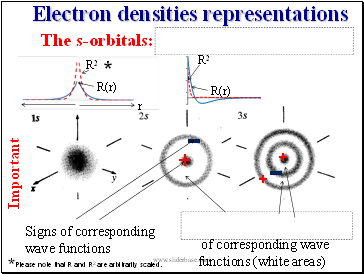## Electron densities representations

Important

+

-

+

+

-

of corresponding wave

functions (white areas)

Signs of corresponding wave functions

R(r)

R2

r

*

*

Please note that R and R2 are arbitrarily scaled.

The s-orbitals:

R2

R(r)

Slide 49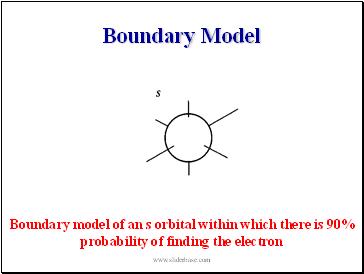Boundary model of an s orbital within which there is 90% probability of finding the electron

Boundary Model

Slide 50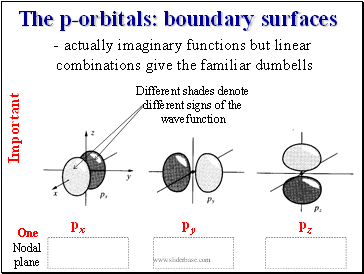The p-orbitals: boundary surfaces

- actually imaginary functions but linear

combinations give the familiar dumbells

px

pz

py

Important

Different shades denote different signs of the wavefunction

One Nodal plane

Slide 51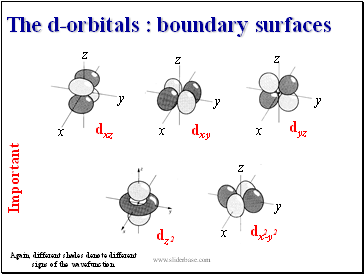The d-orbitals : boundary surfaces

Important

Again, different shades denote different signs of the wavefunction

dxz

dxy

dyz

dxz

dz

2

dx-y

2

2

z

z

z

z

x

x

x

x

y

y

y

y

Slide 52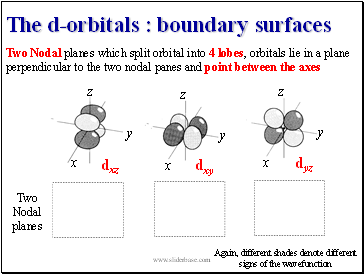The d-orbitals : boundary surfaces

Again, different shades denote different signs of the wavefunction

dxz

dxy

dyz

dzx

Two

Nodal planes

dxz

dxy

dyz

dxz

Two Nodal planes which split orbital into 4 lobes, orbitals lie in a plane perpendicular to the two nodal panes and point between the axes

z

x

y

z

x

y

z

x

y

Slide 53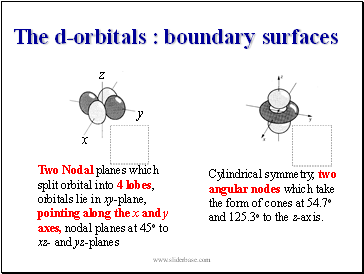The d-orbitals : boundary surfaces

2

2

2

z

x

y

Two Nodal planes which split orbital into 4 lobes, orbitals lie in xy-plane, pointing along the x and y axes, nodal planes at 45o to xz- and yz-planes

Cylindrical symmetry, two angular nodes which take the form of cones at 54.7o and 125.3o to the z-axis.

Slide 54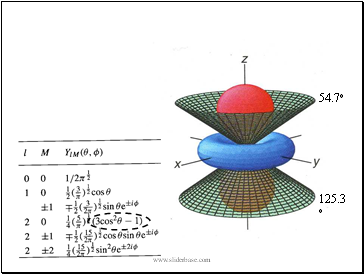54.7o

125.3o

Slide 55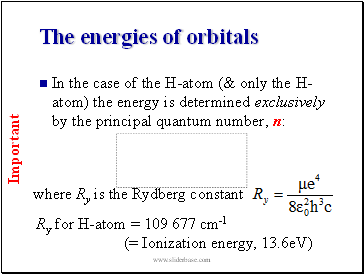## The energies of orbitals

In the case of the H-atom (& only the H-atom) the energy is determined exclusively by the principal quantum number, n:

Important

Slide 56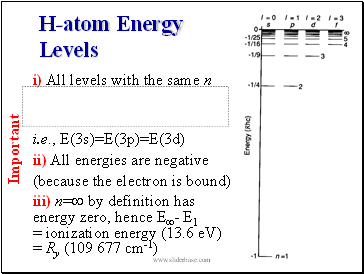H-atom Energy Levels

i) All levels with the same n

i.e., E(3s)=E(3p)=E(3d)

ii) All energies are negative

Go to page:
1  2  3  4  5  6  7  8  9  10  11  12  13  14  15
16  17